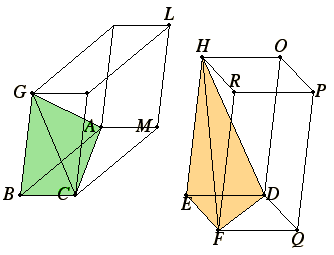# Proposition 9

In equal pyramids with triangular bases the bases are reciprocally proportional to the heights; and those pyramids are equal in which the bases are reciprocally proportional to the heights.

Let there be equal pyramids with triangular bases ABC and DEF and vertices G and H.

I say that in the pyramids ABCG and DEFH the bases are reciprocally proportional to the heights, that is the base ABC is to the base DEF as the height of the pyramid DEFH is to the height of the pyramid ABCG.

Complete the parallelepipedal solids BGML and EHQP.XII.7,Cor

Now, since the pyramid ABCG equals the pyramid DEFH, and the solid BGML is six times the pyramid ABCG, and the solid EHQP six times the pyramid DEFH, therefore the solid BGML equals the solid EHQP.

XI.34

But in equal parallelepipedal solids the bases are reciprocally proportional to the heights, therefore the base BM is to the base EQ as the height of the solid EHQP is to the height of the solid BGML.

But the base BM is to EQ as the triangle ABC is to the triangle DEF. Therefore the triangle ABC is to the triangle DEF as the height of the solid EHQP is to the height of the solid BGML.

But the height of the solid EHQP is identical with the height of the pyramid DEFH, and the height of the solid BGML is identical with the height of the pyramid ABCG, therefore the base ABC is to the base DEF as the height of the pyramid DEFH is to the height of the pyramid ABCG.

Therefore in the pyramids ABCG and DEFH the bases are reciprocally proportional to the heights.

Next, in the pyramids ABCG and DEFH let the bases be reciprocally proportional to the heights, that is, as the base ABC is to the base DEF, so let the height of the pyramid DEFH be to the height of the pyramid ABCG.

I say that the pyramid ABCG equals the pyramid DEFH.

V.11

With the same construction, since the base ABC is to the base DEF as the height of the pyramid DEFH is to the height of the pyramid ABCG, while the base ABC is to the base DEF as the parallelogram BM is to the parallelogram EQ, therefore the parallelogram BM is to the parallelogram EQ as the height of the pyramid DEFH is to the height of the pyramid ABCG.

But the height of the pyramid DEFH is identical with the height of the parallelepiped EHQP, and the height of the pyramid ABCG is identical with the height of the parallelepiped BGML, therefore the base BM is to the base EQ as the height of the parallelepiped EHQP is to the height of the parallelepiped BGML.

XI.34

But those parallelepipedal solids in which the bases are reciprocally proportional to the heights are equal, therefore the parallelepipedal solid BGML equals the parallelepipedal solid EHQP.

And the pyramid ABCG is a sixth part of BGML, and the pyramid DEFH a sixth part of the parallelepiped EHQP, therefore the pyramid ABCG equals the pyramid DEFH.

Therefore, in equal pyramids with triangular bases the bases are reciprocally proportional to the heights; and those pyramids are equal in which the bases are reciprocally proportional to the heights.

Q.E.D.

## Guide

The pyramids with triangular bases are one-third of the prisms with triangular bases, which aren’t drawn, and the prisms are half of the parallelepipeds. Since the analogous proposition XI.34 holds for parallelepipeds, this proposition holds for pyramids.

When a similar situation appears later where cones are one-third of cylinders, Euclid simply says the same holds for cones, too, with no details whatsoever.

This proposition completes the theory of volumes for pyramids. The next few propositions treat the theory of volumes of cones and cylinders.